# 搜索中常见数据结构与算法探究（二）

• 2022-10-12
北京
• 本文字数：6529 字

阅读完需：约 21 分钟### 2 图论基础

#### 2.1 图的基本概念#### 2.2 图的表示方法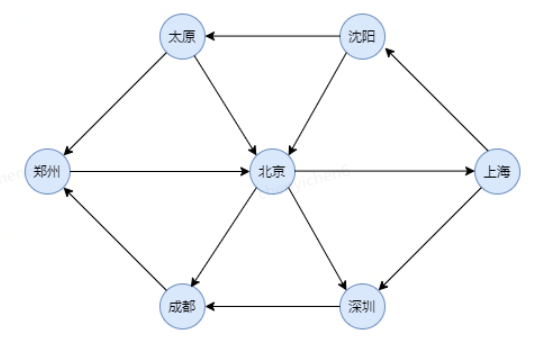1）邻接矩阵

/*** <p/>* Description: 使用邻接矩阵的图表示法* <p/>* Company: <a href=www.jd.com>京东</a>** @author <a href=mailto:pankun8@jd.com>pankun8</a>* @date 2021/11/11 15:41*/@Data@NoArgsConstructorpublic class Graph<T extends Node>{/*** 图的节点数*/private int n;/*** 图*/private T[] data;/*** 是否是有向图*/private Boolean directed;/*** 邻接矩阵*/private int[][] matrix;public Graph(T[] data , Boolean directed){this.n = data.length;this.data = data;this.directed = directed;matrix = new int[n][n];}public void init(T[] data , Boolean directed){this.n = data.length;this.data = data;this.directed = directed;matrix = new int[n][n];}/**** @param v 起点* @param w 终点* @param value 权重*/public void addEdge(int v , int w , int value){if((v >=0 && v < n) && (w >= 0 && w < n)){if(hasEdge(v,w) == value){return;}matrix[v][w] = value;if(!this.directed){matrix[w][v] = value;}n ++;}}//判断两个节点中是否以及存在边public int hasEdge(int v, int w){if((v >=0 && v < n) && (w >= 0 && w < n)){return matrix[v][w];}return 0;}/*** 状态转移函数* @param index* @param value* @return*/public int stateTransfer(int index , int value){int[] matrix = this.matrix[index];for (int i = 0; i < matrix.length; i++) {if(matrix[i] == value){return i;}}return Integer.MAX_VALUE;}}

2）邻接表

#### 2.3 图的搜索算法

• 深度优先搜索算法（DFS）

• 广度优先搜索算法（BFS）

### 3 数据结构与算法

#### 3.1 BF（Brute Force）算法

3.1.1 算法介绍

BF（Brute Force）算法也可以叫暴力匹配算法或者朴素匹配算法。

3.1.2 算法过程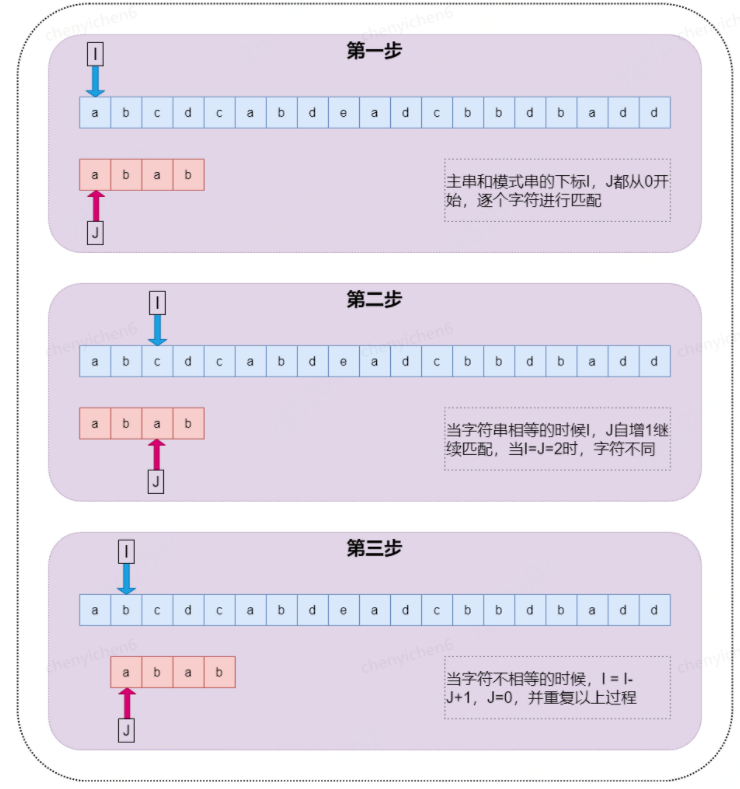3.1.3 算法分析

BF 算法从很“暴力”，当然也就比较简单，好懂，但是响应的性能也不高极端情况下时间复杂度函数为 O(m*n)。

• 朴素字符串匹配算法思想简单，代码实现也非常简单，不容易出错，容易调试和修改。

• 在实际的软件开发中，模式串和主串的长度都不会太长，大部分情况下，算法执行的效率都不会太低。

#### 3.2 RK（Rabin-Karp）算法

3.2.1 算法介绍

RK 算法全程叫 Rabin-Karp 算法，是有它的两位发明者 Rabin 和 Karp 的名字来命名，这个算法理解并不难，他其实是 BF 算法的升级版。

3.2.2 算法过程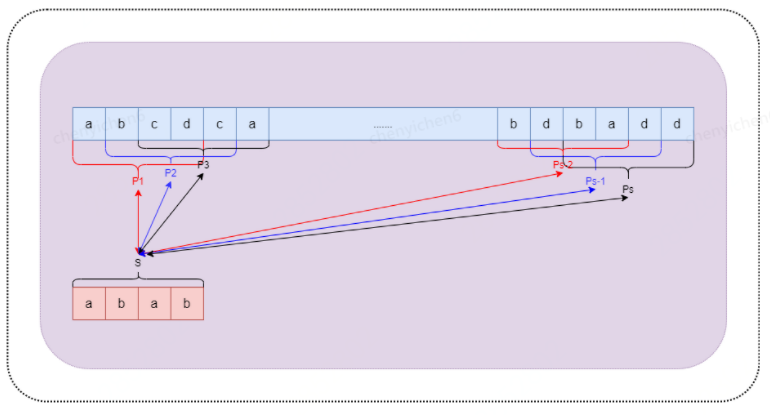3.2.3 算法分析

• 计算模式串哈希和子串的哈希；

• 模式串哈希和子串哈希的比较；

#### 3.3 KMP 算法

3.3.1 算法介绍

KMP 算法是一种线性时间复杂度的字符串匹配算法，它是对 BF(Brute-Force)算法的改进。KMP 算法是由 D.E.Knuth 与 V.R.Partt 和 J.H.Morris 一起发现的，因此人们称它为 Knuth-Morris-Pratt 算法，简称 KMP 算法。

3.3.2 算法过程

• **前缀：**不包含最后一个字符的所有以第一个字符开头的连续子串；

• 后缀：不包含第一个字符的所有以最后一个字符结尾的连续子串；

• 最大公共前后缀：前缀集合与后缀集合中长度最大的子串；

KMP 算法的过程如下图：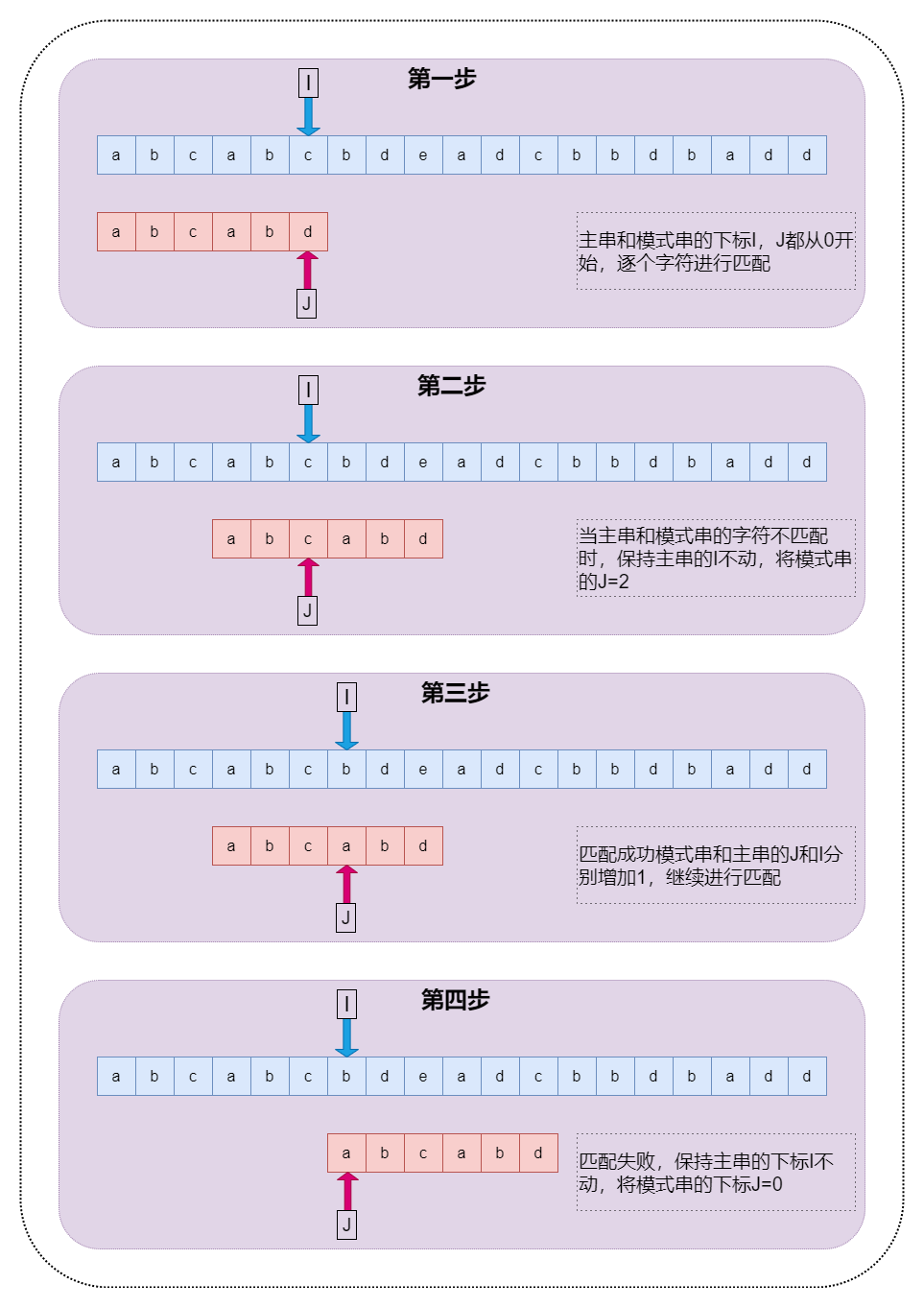public static void genNext(Integer[] next , String p){int j = 0 , k = -1;char[] chars = p.toCharArray();next = -1;while(j < p.length() - 1){if(k == -1 || chars[j] == chars[k]){j++;k++;next[j] = k;}else{k = next[k];//此处为理解难点}}}

1）特殊情况

2）当 P[j]==P[k]的情况

3）当 P[j]!=P[k]的情况

4）算法优化

public void genNext(Integer[] next , String p){int j = 0 , k = -1;char[] chars = p.toCharArray();next = -1;while(j < p.length() - 1){if(k == -1 || chars[j] == chars[k]){j++;k++;if(chars[j] == chars[k]){next[j] = next[k];//如果两个相等}else{next[j] = k;}}else{k = next[k];}}}

3.3.3 算法分析

KMP 算法通过消除主串指针的回溯提高匹配的效率，整个算法分为两部分，next 数据的求解，以及字符串匹配，从上一节的分析可知求解 next 数组的时间复杂度为 O(m)，匹配算法的时间复杂度为 O(n)，整体的时间复杂度为 O(m+n)。KMP 算法不是最快匹配算法，却是名气最大的，使用的范围也非常广。

#### 3.4 BM 算法

3.4.1 算法介绍

Boyer-Moore 字符串搜索算法是一种非常高效的字符串搜索算法。它由 Bob Boyer 和 J Strother Moore 发明，有实验统计它的性能是 KMP 算法的 3-4 倍。

3.4.2 算法过程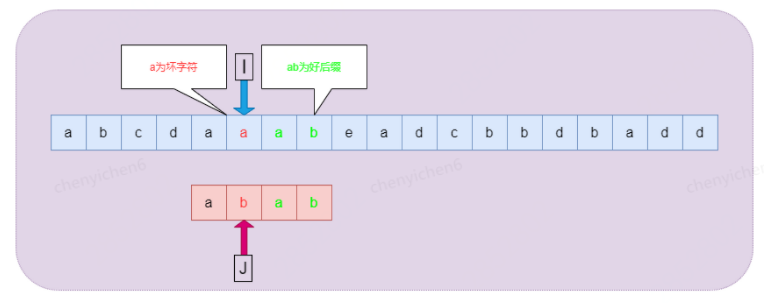1）坏字符规则：在 BM 算法从右向左扫描的过程中，若发现某个字符 S[i]不匹配时，则按照如下两种情况进行处理：

• 如果字符 S[i]在模式串 P 中没有出现，那么从字符 S[i]开始的 m 个文本显然是不可能和 P 匹配成功，直接全部跳过该区域。

• 如果字符 S[i]在模式串 P 中出现，则以该字符进行对齐。

2）好后缀规则：在 BM 算法中，若发现某个字符不匹配的同时，已有部分字符匹配成功，则按照如下两种情况进行处理：

• 如果已经匹配的子串在模式串 P 中出现过，且子串的前一个字符和 P[j]不相同，则将模式串移动到首次出现子串的前一个位置。

• 如果已经匹配的子串在模式串 P 中没有出现过，则找到已经匹配的子串最大前缀，并移动模式串 P 到最大前缀的前一个字符。

BM 算法过程如下：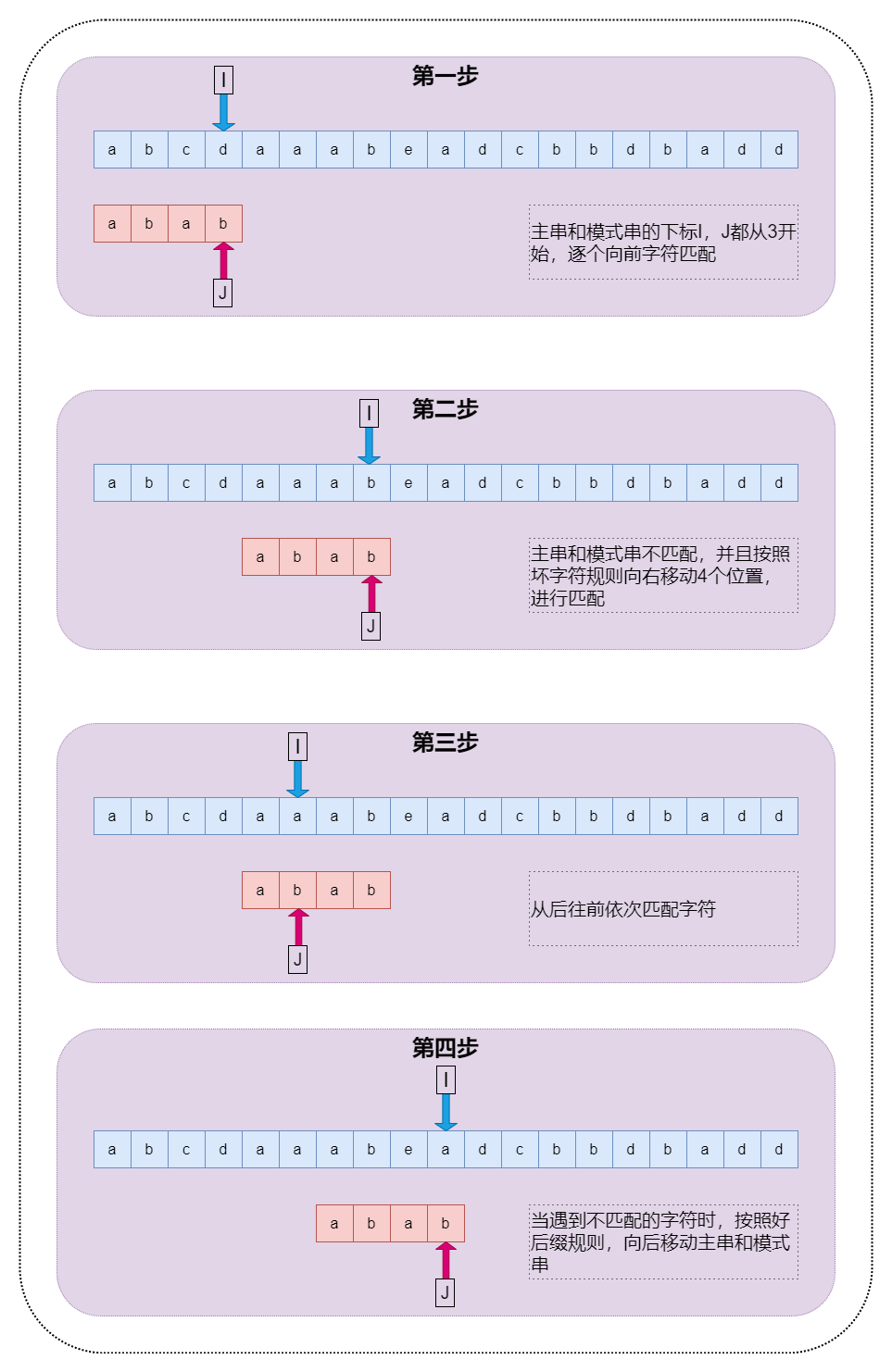3.4.3 算法分析

#### 3.5 TireTree

3.5.1 算法介绍

TireTree 的核心思想是空间换时间。TrieTree 是一种高效的索引方法，它实际上是一种确定有限自动机(DFA)，利用字符串的公共前缀来降低查询时间的开销以达到提高查询效率的目的，非常适合多模式匹配。TireTree 有以下基本性质：

• 根节点不包含字符，除根节点外每个节点都包含一个字符。

• 从根节点到某一个节点，路径上经过的字符连接起来，为该节点对应的字符串。

• 每个节点对应的所有子节点包含的字符都不相同。

3.5.2 算法过程

TireTree 构建与查询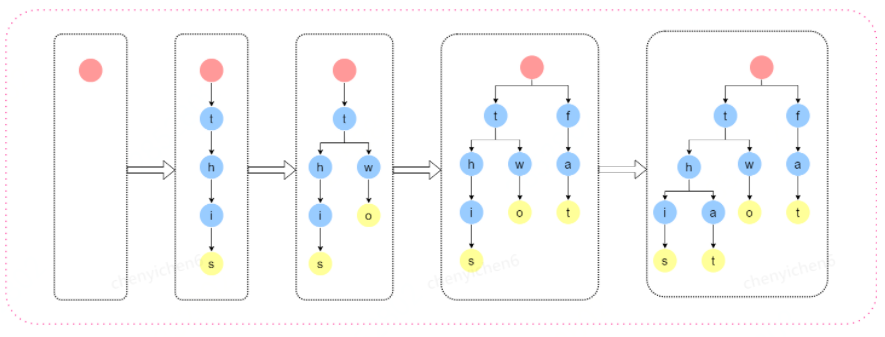#### 3.5.3 算法分析

TireTree 由于本身的特性非常适合前缀查找个普通查找，并且查询的时间复杂度为 O(log(n))，和 hash 比较在一些场景下性能要优于甚至取代 hash，例如说前缀查询（hash 不支持前缀查询）。

#### 3.6 AC 自动机

3.6.1 算法介绍

AC 自动机（Aho-Corasick automation）该算法在 1975 年产生于贝尔实验室，是著名的多模匹配算法之一。要搞懂 AC 自动机，先得有 TireTree 和 KMP 模式匹配算法的基础知识，上述章节有 TireTree 和 KMP 算法的详细介绍。

3.6.2 算法过程

AC 自动机的构建过程需要如下步骤：

• TireTree 的构建，请参看 TireTree 章节

• fail 指针的构建 - 使当前字符失配时跳转到具有最长公共前后缀的字符继续匹配。如同 KMP 算法一样， AC 自动机在匹配时如果当前字符匹配失败，那么利用 fail 指针进行跳转。由此可知如果跳转，跳转后的串的前缀，必为跳转前的模式串的后缀并且跳转的新位置的深度一定小于跳之前的节点。fail 指针的求解过程可是完全参照 KMP 算法的 next 指针求解过程，此处不再赘述。

• AC 自动机查找 - 查找过程和 TireTree 相同，只是在查找失败的时候感觉 fail 指针跳转到指定的位置继续进行匹配。

3.6.3 算法分析

AC 自动机利用 fail 指针阻止了模式串匹配阶段的回溯，将时间复杂度优化到了 O(n)。

#### 3.7 Double-Array-TireTree

3.7.1 算法介绍

3.7.2 算法过程

Double-Array-TireTree 构建

Double-Array-TireTree 终究是一个树结构，树结构的两个重要的要素便是前驱和后继，把树压缩在双数组中，只需要保持能查到每个节点的前驱和后继。首先要介绍几个重要的概念：

• STATE：状态，实际是在数组中的下标

• CODE：状态转移值，实际为转移字符的值

• BASE：标识后继节点的基地址数组

• CHECK：标识前驱节点的地址

• state[t] = base[state[s]] + code[c]

• check[state[t]] = state[s]

• 动态输入词语，动态构建双数组

• 已知所有词语，静态构建双数组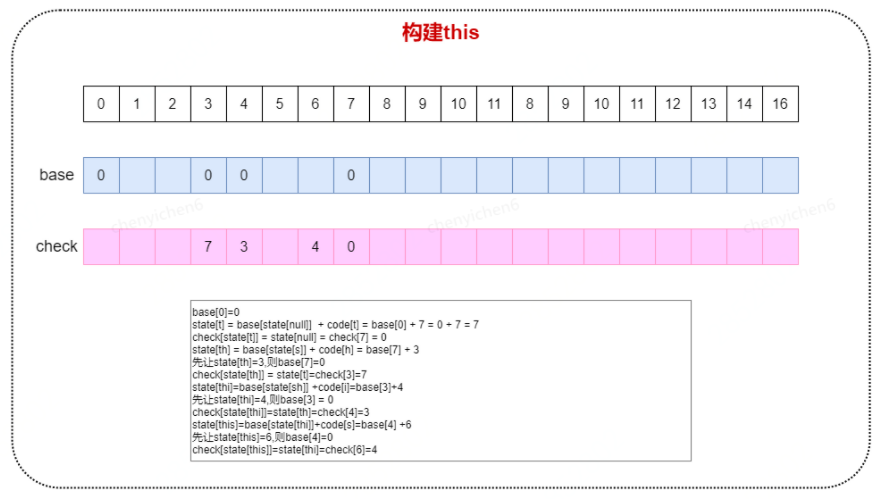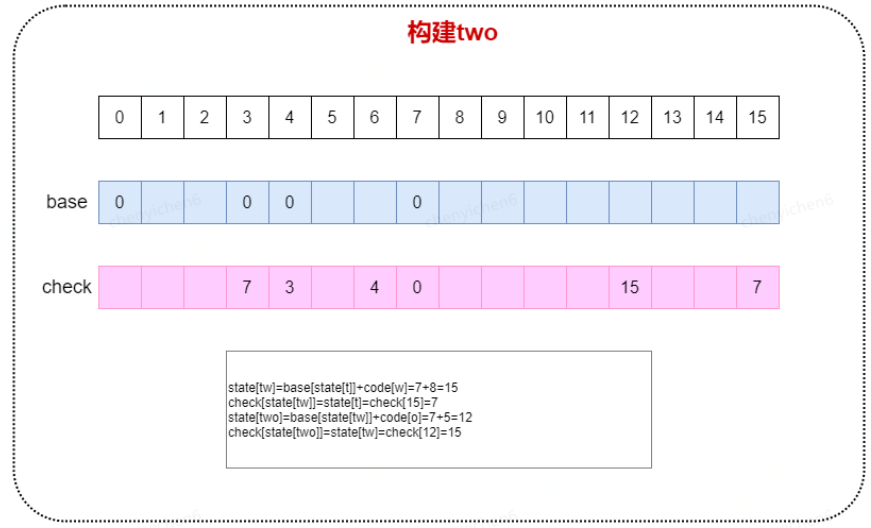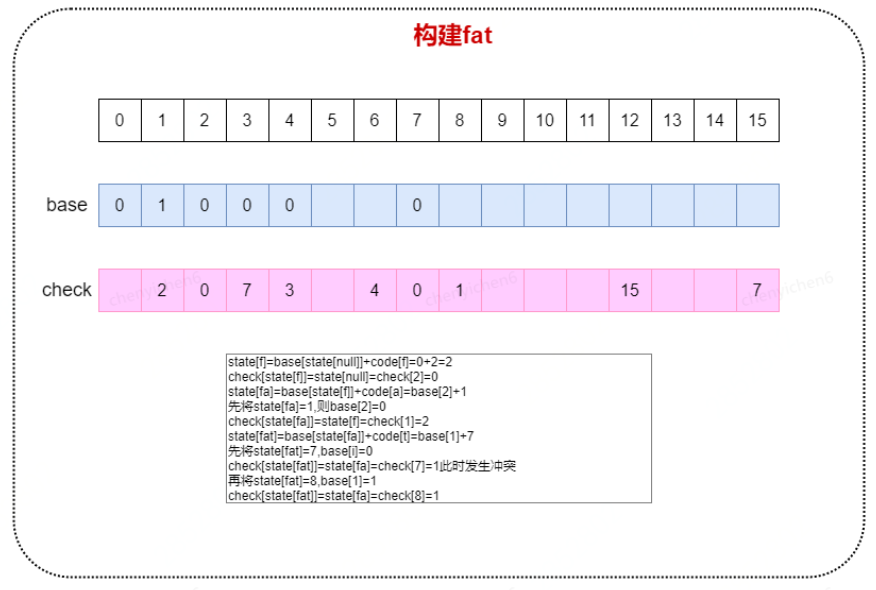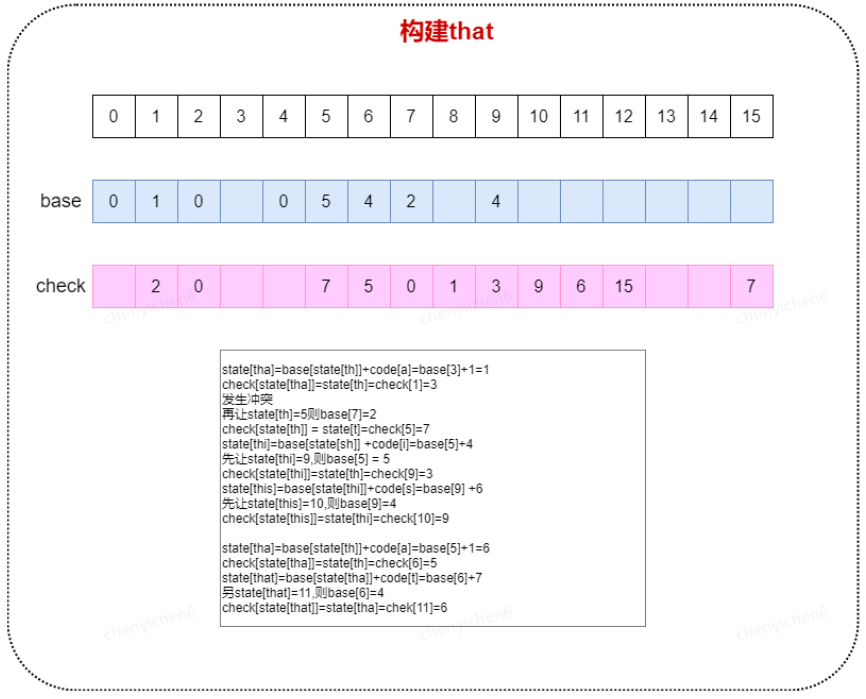Double-Array-TireTree 查询

1）state[t] = base[state[null]]+code[t]= 0 + 7=7

check=state[null]=0 通过

2）state[th] = base[state[t]]+code[h]=base+3 =2+3=5

check= state[t] = 7 通过

3）state[tha] = base[state[th]]+ code[a]=base+1=5+1=6

check=state[th]=5 通过

4）state[that] = base[state[tha]]+t = base+7=11

check=state[tha]=6 通过

3.7.3 算法分析

### 4 参考资料

《数据结构与算法分析：java 语言描述》

《自动机理论、语言和计算导论》## 评论